# Python – Data visualization using Bokeh

Bokeh is a data visualization library in Python that provides high-performance interactive charts and plots. Bokeh output can be obtained in various mediums like notebook, html and server. It is possible to embed bokeh plots in Django and flask apps.

Bokeh provides two visualization interfaces to users:

bokeh.models : A low level interface that provides high flexibility to application developers.
bokeh.plotting : A high level interface for creating visual glyphs.

To install bokeh package, run the following command in the terminal:

`pip install bokeh`

The dataset used for generating bokeh graphs is collected from Kaggle.

Code #1: Scatter Markers
To create scatter circle markers, circle() method is used.

 `# import modules ` `from` `bokeh.plotting ``import` `figure, output_notebook, show ` ` `  `# output to notebook ` `output_notebook() ` ` `  `# create figure ` `p ``=` `figure(plot_width ``=` `400``, plot_height ``=` `400``) ` ` `  `# add a circle renderer with ` `# size, color and alpha ` `p.circle([``1``, ``2``, ``3``, ``4``, ``5``], [``4``, ``7``, ``1``, ``6``, ``3``],  ` `         ``size ``=` `10``, color ``=` `"navy"``, alpha ``=` `0.5``) ` ` `  `# show the results ` `show(p)  `

Output :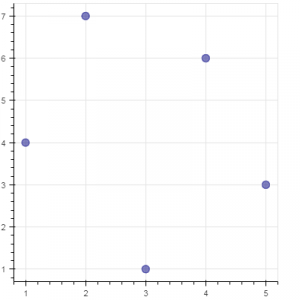Code #2: Single line
To create a single line, line() method is used.

 `# import modules ` `from` `bokeh.plotting ``import` `figure, output_notebook, show ` ` `  `# output to notebook ` `output_notebook() ` ` `  `# create figure ` `p ``=` `figure(plot_width ``=` `400``, plot_height ``=` `400``) ` `  `  `# add a line renderer ` `p.line([``1``, ``2``, ``3``, ``4``, ``5``], [``3``, ``1``, ``2``, ``6``, ``5``],  ` `        ``line_width ``=` `2``, color ``=` `"green"``) ` ` `  `# show the results ` `show(p) `

Output :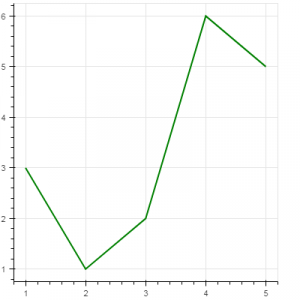Code #3: Bar Chart
Bar chart presents categorical data with rectangular bars. The length of the bar is proportional to the values that are represented.

 `# import necessary modules ` `import` `pandas as pd ` `from` `bokeh.charts ``import` `Bar, output_notebook, show ` ` `  `# output to notebook ` `output_notebook() ` ` `  `# read data in dataframe ` `df ``=` `pd.read_csv(r``"D:/kaggle/mcdonald/menu.csv"``) ` ` `  `# create bar ` `p ``=` `Bar(df, ``"Category"``, values ``=` `"Calories"``, ` `        ``title ``=` `"Total Calories by Category"``,  ` `                        ``legend ``=` `"top_right"``) ` ` `  `# show the results ` `show(p) `

Output :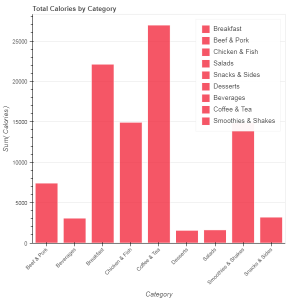Code #4: Box Plot
Box plot is used to represent statistical data on a plot. It helps to summarize statistical properties of various data groups present in the data.

 `# import necessary modules ` `from` `bokeh.charts ``import` `BoxPlot, output_notebook, show ` `import` `pandas as pd ` ` `  `# output to notebook ` `output_notebook() ` ` `  `# read data in dataframe ` `df ``=` `pd.read_csv(r``"D:/kaggle / mcdonald / menu.csv"``) ` ` `  `# create bar ` `p ``=` `BoxPlot(df, values ``=` `"Protein"``, label ``=` `"Category"``,  ` `            ``color ``=` `"yellow"``, title ``=` `"Protein Summary (grouped by category)"``, ` `             ``legend ``=` `"top_right"``) ` ` `  `# show the results ` `show(p) `

Output :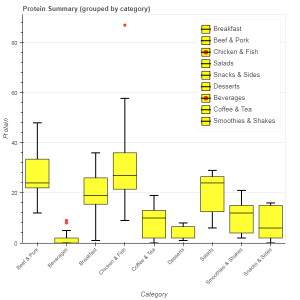Code #5: Histogram
Histogram is used to represent distribution of numerical data. The height of a rectangle in a histogram is proportional to the frequency of values in a class interval.

 `# import necessary modules ` `from` `bokeh.charts ``import` `Histogram, output_notebook, show ` `import` `pandas as pd ` ` `  `# output to notebook ` `output_notebook() ` ` `  `# read data in dataframe ` `df ``=` `pd.read_csv(r``"D:/kaggle / mcdonald / menu.csv"``) ` ` `  `# create histogram ` `p ``=` `Histogram(df, values ``=` `"Total Fat"``, ` `               ``title ``=` `"Total Fat Distribution"``,  ` `               ``color ``=` `"navy"``) ` ` `  `# show the results ` `show(p)  `

Output :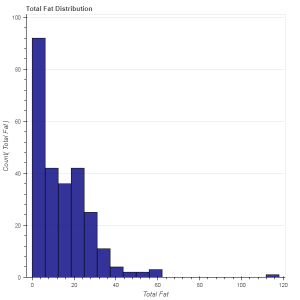Code #6: Scatter plot
Scatter plot is used to plot values of two variables in a dataset. It helps to find correlation among the two variables that are selected.

 `# import necessary modules ` `from` `bokeh.charts ``import` `Scatter, output_notebook, show ` `import` `pandas as pd ` ` `  `# output to notebook ` `output_notebook() ` ` `  `# read data in dataframe ` `df ``=` `pd.read_csv(r``"D:/kaggle / mcdonald / menu.csv"``) ` ` `  `# create scatter plot ` `p ``=` `Scatter(df, x ``=` `"Carbohydrates"``, y ``=` `"Saturated Fat"``, ` `            ``title ``=` `"Saturated Fat vs Carbohydrates"``, ` `            ``xlabel ``=` `"Carbohydrates"``, ylabel ``=` `"Saturated Fat"``, ` `            ``color ``=` `"orange"``) ` `  `  `# show the results ` `show(p)  `

Output :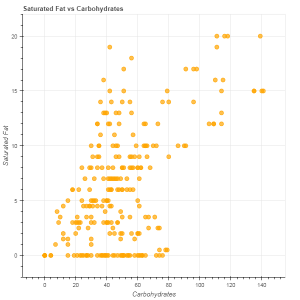References: https://bokeh.pydata.org/en/latest/

Attention geek! Strengthen your foundations with the Python Programming Foundation Course and learn the basics.

To begin with, your interview preparations Enhance your Data Structures concepts with the Python DS Course.

My Personal Notes arrow_drop_upCheck out this Author's contributed articles.

If you like GeeksforGeeks and would like to contribute, you can also write an article using contribute.geeksforgeeks.org or mail your article to contribute@geeksforgeeks.org. See your article appearing on the GeeksforGeeks main page and help other Geeks.

Please Improve this article if you find anything incorrect by clicking on the "Improve Article" button below.

Article Tags :

7

Please write to us at contribute@geeksforgeeks.org to report any issue with the above content.